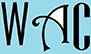## Solving circumference, radius, diameter, perimeter, height, & triangles

This Webpage is for disadvantaged students in special education programs & special education with special needs and college students who need help with strategies in geometry in order to successfully answer geometry questions in solving circumference, radius, diameter, perimeter, height, and triangle. These are popular geometry questions that they can work on or refer to in order to do their geometry homework or answer geometry problems on their geometry worksheets.

We provide geometry online tutor for high school and college students, especially those from low income backgrounds, to help them with their geometry homework online.  We have provided geometry online questions and answers for circumference, radius, diameter, perimeter, height, and triangle problems to assist students with their geometry practice, geometry study, geometry homework, and geometry tests and quizzes.

Geometry definition: Geometry is a form of math that utilizes angles and sides of shape to solve real world or engineering issues.  These questions below also have answer for the geometry online problems when you click on each link.  Here are a list of the most popular and most common geometry online problems that can be found in many geometry homework and geometry exams.  The answers for the geometry online problems are explained in steps and the math answers are easily understood for circumference, radius, diameter, perimeter, height, and triangle questions.